GFG App
Open AppBrowser
Continue

# Predicting Stock Price Direction using Support Vector Machines

We are going to implement an End-to-End project using Support Vector Machines to live Trade For us. You Probably must have Heard of the term stock market which is known to have made the lives of thousands and to have destroyed the lives of millions. If you are not familiar with the stock market you can surf some basic Stuff about markets.

## Tools and Technologies Used :

• Python
• Sklearn- Support Vector Classifier
• Yahoo Finance
• Jupyter-Notebook
• BlueShift

## Python3

 `# Machine learning ` `from` `sklearn.svm ``import` `SVC ` `from` `sklearn.metrics ``import` `accuracy_score ` ` `  `# For data manipulation ` `import` `pandas as pd ` `import` `numpy as np ` ` `  `# To plot ` `import` `matplotlib.pyplot as plt ` `plt.style.use(``'seaborn-darkgrid'``) ` ` `  `# To ignore warnings ` `import` `warnings ` `warnings.filterwarnings(``"ignore"``)`

### Step 2: Read Stock  data

We will Read the Stock Data Downloaded From Yahoo Finance Website. The Data Is stored in OHLC(Open, High, Low, Close)  format in a CSV file. To read a CSV file, you can use the read_csv() method of pandas.

Syntax :

`pd.read_csv(filename, index_col)`

Note: We have downloaded the past 1 year data of Reliance Industries Trading In NSE from Yahoo Finance Website.

File Used:

## Python3

 `# Read the csv file using read_csv  ` `# method of pandas ` `df ``=` `pd.read_csv(``'RELIANCE.csv'``) ` `df`

Output: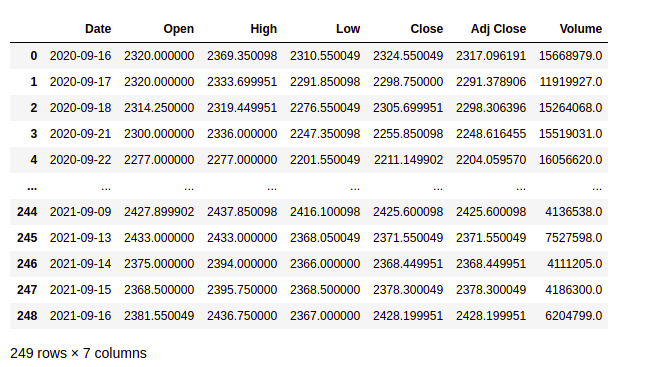### Step 3: Data Preparation

The data needed to be processed before use such that the date column should act as an index to do that

## Python3

 `# Changes The Date column as index columns ` `df.index ``=` `pd.to_datetime(df[``'Date'``]) ` `df ` ` `  `# drop The original date column ` `df ``=` `df.drop([``'Date'``], axis``=``'columns'``) ` `df `

Output: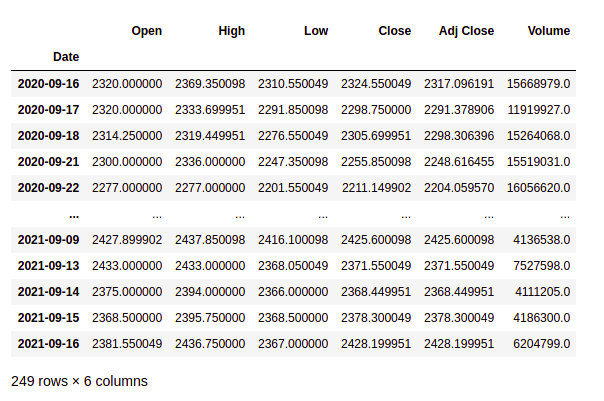### Step 4: Define the explanatory variables

Explanatory or independent variables are used to predict the value response variable. The X is a dataset that holds the variables which are used for prediction. The X consists of variables such as ‘Open – Close’ and ‘High – Low’. These can be understood as indicators based on which the algorithm will predict tomorrow’s trend. Feel free to add more indicators and see the performance

## Python3

 `# Create predictor variables ` `df[``'Open-Close'``] ``=` `df.``Open` `-` `df.Close ` `df[``'High-Low'``] ``=` `df.High ``-` `df.Low ` ` `  `# Store all predictor variables in a variable X ` `X ``=` `df[[``'Open-Close'``, ``'High-Low'``]] ` `X.head() `

Output: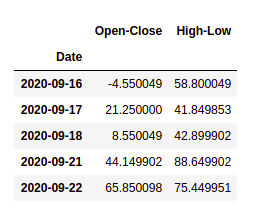### Step 5: Define the target variable

The target variable is the outcome which the machine learning model will predict based on the explanatory variables. y is a target dataset storing the correct trading signal which the machine learning algorithm will try to predict. If tomorrow’s price is greater than today’s price then we will buy the particular Stock else we will have no position in the. We will store +1 for a buy signal and 0 for a no position in y. We will use where() function from NumPy to do this.

Syntax:

`np.where(condition,value_if_true,value_if_false)`

## Python3

 `# Target variables ` `y ``=` `np.where(df[``'Close'``].shift(``-``1``) > df[``'Close'``], ``1``, ``0``) ` `y`

Output: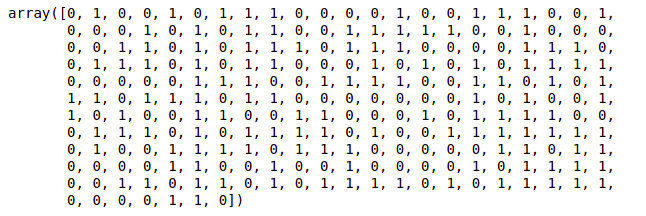### Step 6: Split the data into train and test

We will split data into training and test data sets. This is done so that we can evaluate the effectiveness of the model in the test dataset

## Python3

 `split_percentage ``=` `0.8` `split ``=` `int``(split_percentage``*``len``(df)) ` ` `  `# Train data set ` `X_train ``=` `X[:split] ` `y_train ``=` `y[:split] ` ` `  `# Test data set ` `X_test ``=` `X[split:] ` `y_test ``=` `y[split:]`

### Step 7: Support Vector Classifier (SVC)

We will use SVC() function from sklearn.svm.SVC library to create our classifier model using the fit() method on the training data set.

## Python3

 `# Support vector classifier ` `cls` `=` `SVC().fit(X_train, y_train)`

### Step 8: Classifier accuracy

We will compute the accuracy of the algorithm on the train and test the data set by comparing the actual values of the signal with the predicted values of the signal. The function accuracy_score() will be used to calculate the accuracy.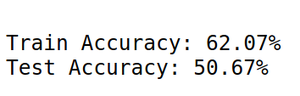An accuracy of 50%+ in test data suggests that the classifier model is effective.

### Step 9: Strategy implementation

We will predict the signal (buy or sell) using the cls.predict() function.

## Python3

 `df[``'Predicted_Signal'``] ``=` `cls``.predict(X)`

Calculate Daily returns

## Python3

 `# Calculate daily returns ` `df[``'Return'``] ``=` `df.Close.pct_change()`

Calculate Strategy Returns

## Python3

 `# Calculate strategy returns ` `df[``'Strategy_Return'``] ``=` `df.Return ``*``df.Predicted_Signal.shift(``1``)`

Calculate Cumulative Returns

## Python3

 `# Calculate Cumulutive returns ` `df[``'Cum_Ret'``] ``=` `df[``'Return'``].cumsum() ` `df`

Calculate Strategy Cumulative Returns

## Python3

 `# Plot Strategy Cumulative returns  ` `df[``'Cum_Strategy'``] ``=` `df[``'Strategy_Return'``].cumsum() ` `df`

## Python3

 `import` `matplotlib.pyplot as plt ` `%``matplotlib inline ` ` `  `plt.plot(Df[``'Cum_Ret'``],color``=``'red'``) ` `plt.plot(Df[``'Cum_Strategy'``],color``=``'blue'``)`

Output: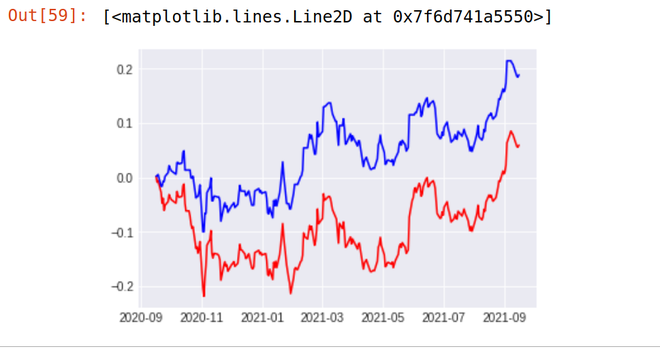As You Can See Our Strategy Seem to be Totally Outperforming the Performance of The Reliance Stock. Our Strategy(Blue Line) Provided the return of 18.87 % in the last 1 year whereas the stock of Reliance Industries (Red Line) Provides the Return of just 5.97%  in the last 1 year.

## Back-testing Result

1.  TCS

```Stock Return Over Last 1 year -  48%
Strategy result - 48.9 %```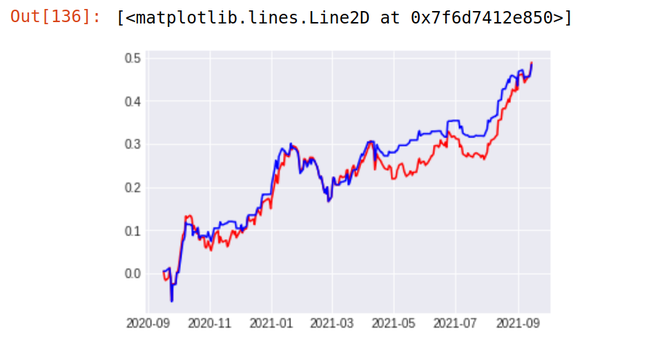2. ICICI BANK

```Stock Return Over Last 1 year -  48%
Strategy result - 48.9 %```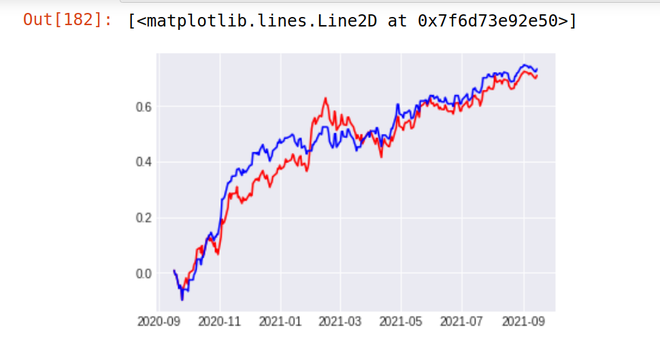## Deploying Strategy To Live Market

The Strategy Coded Can be easily deployed in the live market and can also be back-tested on any number of data throughout exchanges. The deployment can be easily done Using the BlueShift Platform. It is an Interactive Platform with Live Data Feed and connections through various Brokers. You can Do Back-testing On the BlueShift platform for any Number Of Time with data from various Exchanges.

## Conclusion

• The Strategy Provider Promising Returns During Live market. Currently, I have just trained the model based on previous day levels however to increase the accuracy of the model we also add various Technical Indicators for training the model such as RSI, ADX,  ATR, MACD, Stochastic, and Many more.
• To Get More Accuracy in Live Market Deep Learning Proved to be Very Effective In Trading in a live market.  We can Automate our Trades using Reinforcement Learning and also using Stacked LSTM which gives exponential Rise for our Strategy returns.

Note: Real Money Should not be deployed until complete Backtesting of Strategy and without promising returns by Strategy during Paper-Trading

My Personal Notes arrow_drop_up